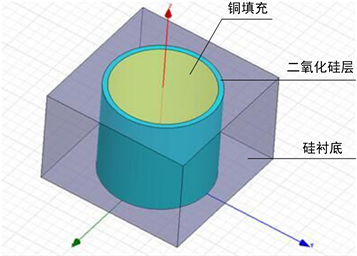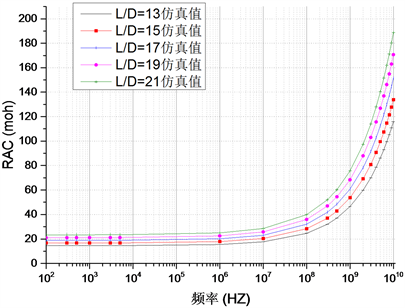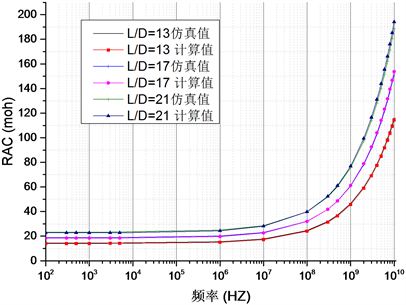#### 期刊菜单

Study on Extracting Analytical Formula for TSV Resistance Parameter Calculation in 3D IC
DOI: 10.12677/OJCS.2020.93008, PDF , HTML, XML, 下载: 572  浏览: 931

Abstract: TSV technology is the key factor of realizing interlayer vertical interconnection in 3D IC and it brings such advantages to 3D IC as short wiring, small size, low power consumption and heterogeneous feature, etc. As the interconnected TSV in 3D IC, its parasitic parameter extraction will directly in-fluence IC performance in power consumption, time delay and noise, etc. Therefore, extracting TSV parasitic parameter is significant for successful design of a high-performance chip. This paper uses the cylinder TSV with high surface ratio as study subject. By simulating cylinder TSV of different dimension parameters, we obtain a resistance parameter value. The high-precision analytical formula for resistance parameter extraction is derived from electromagnetic field theory and curve fitting method. The analytic formula is able to rapidly and precisely calculate TSV resistance parameter value to largely improve parameter extraction efficiency.

1. 引言Figure 1. Model of cylindrical through silicon via

2. 圆柱硅通孔建模Figure 2. Simulation results of resistance and inductance of a cylindrical through silicon via with high aspect ratio

3. 电阻参数提取解析式

3.1. 公式推导

$R\left(f\right)=\left(R{}_{1\text{GHz}}-{R}_{DC}\right)\sqrt{\frac{f}{{f}_{1\text{GHz}}}}+{R}_{DC}$ (1)

${R}_{DC}=\frac{1}{\sigma }\frac{L}{\pi {r}^{2}}$ (2)

$\sigma$ 为硅通孔中金属的电导率，L为硅通孔高度，r为硅通孔半径。

${R}_{1\text{GHz}}$ 是频率为1 GHz时的电阻值，根据公式(1)可知，要想求出任意频率下的电阻值，需要先对频率为1 GHZ时的电阻值以及直流电阻进行求解，对于 ${R}_{1\text{GHz}}$ 的求解如下。

$\nabla ×\nabla ×E=\nabla ×\left(-\mu \frac{\partial H}{\partial t}\right)=-\mu \frac{\partial }{\partial t}\left(\nabla ×H\right)$ (3)

${\nabla }^{2}E=\nabla \left(\nabla \cdot E\right)-\nabla ×\nabla ×E=\mu \frac{\partial }{\partial t}\left(\sigma E+\frac{\partial \left(\epsilon E\right)}{\partial t}\right)$ (4)

$E={E}_{\text{0}}{\text{e}}^{j\omega t}$ (5)

${\nabla }^{2}E=j\omega \mu \sigma E-{\omega }^{2}\mu \sigma \epsilon E$ (6)

$j\omega \mu {J}_{z}\left(r\right)=\frac{1}{r}\frac{\text{d}}{\text{d}r}\left(r\frac{\text{d}}{\text{d}r}{E}_{z}\left(r\right)\right)$ (7)

${J}_{z}\left(r\right)={c}_{1}{I}_{0}\left(\frac{\left(1+j\right)r}{\delta }\right)$ (8)

$\delta =\sqrt{\frac{\text{1}}{\pi f{\mu }_{0}\sigma }}$ (9)

$\frac{{c}_{1}}{I}=\left(1+j\right){\left[2\pi r\delta {I}_{1}\left(\left(1+j\right)r/\delta \right)\right]}^{-1}$ (10)

${J}_{z}\left(r\right)=\frac{1}{I}\frac{c}{2\pi {r}_{z}{I}_{1}\left(c{r}_{z}\right)}{I}_{0}\left(c{r}_{z}\right)$ (11)

$c=\sqrt{j\omega \mu \sigma }=\frac{1+j}{\delta }$ (12)

$R=\frac{{\int }_{\text{0}}^{L}{\int }_{\text{0}}^{r}{|{J}_{z}|}^{2}\cdot 2\pi r\text{d}r\text{d}z}{{I}^{2}\sigma }$ (13)

$R={\int }_{\text{0}}^{L}\frac{\text{1}}{\text{2}\pi {r}_{z}\sigma }\mathrm{Re}\frac{c{I}_{0}\left(c{r}_{z}\right)}{{I}_{1}\left(c{r}_{z}\right)}\text{d}z$ (14)

$R=\frac{1}{\sigma }\frac{L}{\pi \left[{r}^{2}-{\left(r-\delta \right)}^{2}\right]}$ (15)

$\alpha ={a}_{1}{D}^{{a}_{2}}\cdot \mathrm{ln}\frac{L}{D}+{\alpha }_{3}{D}^{{a}_{4}}$ (16)

$\alpha =0.2652{D}^{0.2831}\cdot \mathrm{ln}\frac{L}{D}+2.9435{D}^{-0.269}$ (17)

${R}_{\text{1GHz}}=\alpha \cdot \frac{1}{\sigma }\cdot \frac{L}{\pi \left[{r}^{2}-{\left(r-\delta \right)}^{2}\right]}$ (18)

3.2. 解析式提取参数结果对比Figure 3. Comparison of resistance values with simulation values obtained by means of formula

4. 结论

  邱碧秀. 微系统封装原理与技术[M]. 北京: 电子工业出版社, 2006.  Guarini, K.W. and TOPol, A.W. (2002) Electrical Integrity of State-of-the-Art 0.13 um SOI CMOS Devices and Circuits Transferred for Three-Dimensional (3D) Integrated Circuit (IC) Fabrication. The International Electron Devices Meeting, Hong Kong, 22 June 2002, 943-945.  Sakuma, K., Andry, P.S. and Tsang, C.K. (2008) 3D Chip-Stacking Technology with Through-Silicon Vias and Low-Volume Lead-Free Interconnections. IBM Journal of Research and Development, 52, 614-622. https://doi.org/10.1147/JRD.2008.5388567  Motoyoshi, M. (2009) Through-Silicon Via (TSV). Proceedings of the IEEE, 97, 43-48. https://doi.org/10.1109/JPROC.2008.2007462  Koyanagi, M. and Fukushima, T. (2009) High-Density through Silicon Vias for 3-D LSIs. Proceedings of the IEEE, 97, 49-59. https://doi.org/10.1109/JPROC.2008.2007463  Pavlidis, V.F. and Friedman, E.G. (2009) Interconnect-Based Design Methodologies for Three-Dimensional Integrated Circuits. Proceedings of the IEEE, 97, 123-139. https://doi.org/10.1109/JPROC.2008.2007473  Wong, B.P., Anurang, M. and Cao, Y. (2004) Nano-Cmos Circuit and Physical Design. John Wiley & Sons, New York. https://doi.org/10.1002/0471653829  Micael, J.T. and Jin, K. (1991) A Hybrid Method for the Calculation of the Resistance and Inductance of Transmission Lines with Arbitrary Cross Sections. Microwave Theory and Techniques, 39, 1338-1342. https://doi.org/10.1109/22.85409  Clayton, R.P. (1994) Analysis of Multiconductor Transmission Lines. John Wiley & Sons, New York.  Khalil, D.E., Ismail, Y., Khellah, M. and Karnik, T. (2008) Analytical Model for the Propagation Delay of Through Silicon Vias. 9th International Symposium on Quality Electronic Design, San Jose, CA, 17-19 March 2008, 553-556. https://doi.org/10.1109/ISQED.2008.4479795  Savidis, I. and Friedman, E.G. (2009) Closed-Form Expres-sions of 3-D Via Resistance, Inductance and Capacitance. IEEE Transactions on Electron Devices, 56, 1873-1881. https://doi.org/10.1109/TED.2009.2026200  Liang, Y.J. and Li, Y. (2011) Closed-Form Expressions for the Resistance and the Inductance of Different Profiles of Through-Silicon Vias. IEEE Electron Device Letters, 32, 393-395. https://doi.org/10.1109/LED.2010.2099203  Khaled, S., Ragai, H., Amin, K., et al. (2011) Compact Lumped Element Model for TSV in 3D-ICS. IEEE, 2321-2324.  Salah, K., El Rouby, A., Ragai, H., et al. (2012) A Closed Form Expression for TSV Based on Chip Spiralinductor. 2012 IEEE International Symposium on Circuits and Systems (ISCAS), Seoul, 20-23 May 2012, 2325-2328. https://doi.org/10.1109/ISCAS.2012.6271760